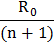# To decrease the range of an ammeter, its resistance need to be increased. An ammeter has resistanceand range I. Which of the following resistance can be connected in series with it to decreases its range to? a)b)c)d) None of these

## Question ID - 150247 :- To decrease the range of an ammeter, its resistance need to be increased. An ammeter has resistanceand range I. Which of the following resistance can be connected in series with it to decreases its range to? a)b)c)d) None of these

3537

 (d) A galvanometer can be converted into an ammeter by using a low resistance wire in parallel with the galvanometer. The range of ammeter can be increased but cannot be decreased. The reason is that a series resistor cannot change the current that will produce full deflection. A shunt connected in parallel is given byIf, then the value of S is negative. Hence, a galvanometer cannot be converted into an ammeter of range.